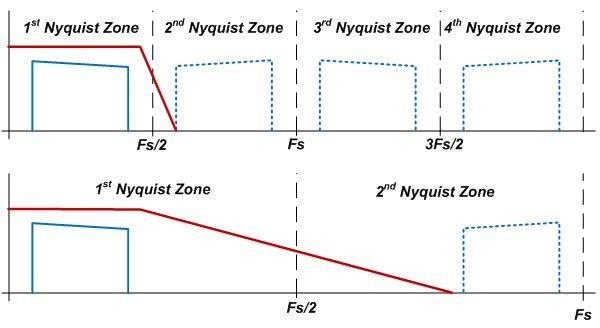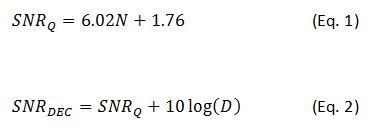# RF sampling: How over-sampling is cheating physics

** This is the fifth post in a new RF-sampling blog series that’ll appear monthly on Analog Wire **

RF sampling converters can capture high-frequency signals and large-bandwidth signals; however, not every application utilizes signals that require very high-speed sampling. For cases where the bandwidth or the output frequency is not excessive, there is still an advantage to utilizing the high sampling rate capabilities of RF sampling converters.

The sampling theorem states that the sampling rate must be at least twice the largest bandwidth of the signal. Sampling below this rate is called under-sampling and causes aliasing; the benefits of this approach were discussed in my previous blog. Sampling above this rate is called over-sampling. Over-sampling offers some processing advantages that seemingly let you defy physics.

One of the key measurement parameters for analog-to-digital converters (ADCs) is signal-to-noise ratio (SNR). SNR measures the relative level between the desired signal power and the entirety of the noise power within the first Nyquist zone. The Nyquist zone bandwidth is the sampling rate divided by two (Fs/2). Recall that all signals and noise will fold back into the first Nyquist zone. This zone effectively represents the entire bandwidth of the device.

One benefit of over-sampling is that the image components are separated farther in frequency space. This allows easier analog filtering to eliminate interfering signals that can alias down into the captured bandwidth and desensitize the receiver. Figure 1 illustrates two cases: one signal sampled near the Nyquist rate and one that is over-sampled. The over-sampled case provides a more realizable analog anti-aliasing filter.Figure 1: Filter impact for Nyquist rate sampling vs. over-sampling

Over-sampling can improve the SNR performance of the device beyond the theoretical quantization noise limitations. The quantization noise is equally distributed across the Nyquist bandwidth. By increasing the sampling rate, the same quantization noise is spread over a larger Nyquist bandwidth. The desired signal remains fixed. Decimation coupled with digital filtering decrease the noise bandwidth without impacting the desired signal. Note, decimation implies over-sampling since there must be additional samples available to remove. In RF sampling ADCs, it is more common to refer to a decimation factor rather than an over-sampling rate; however, these parameters are effectively equivalent.

For example, decimating by two must have the signal over-sampled by at least two. In this example, the signal power remains the same but the Nyquist bandwidth is cut in half. This eliminates half of the noise power, which improves the ADC SNR by 3 dB. The first equation represents the ideal SNR due to quantization noise where N is the number of bits of the converter. The second equation represents the SNR improvement related to the decimation factor D.From a pure quantization noise analysis, each fourfold increase in sampling rate equates to one effective bit of resolution improvement. In theory, a 12-bit data converter can achieve the SNR performance of a 14-bit converter by sampling at 16 times the minimum Nyquist rate. In practice, RF sampling data converters do not achieve SNR performance equivalent to the quantization noise limit due to other impairments related to aperture jitter, clock jitter and thermal noise; however, the over-sampling technique still provides nearly the same relative SNR improvement. In many communication systems, this benefit is critical. For example, the ADS54J60 is a 16-bit, 1-GSPS ADC that has options for decimation by two or four. The designer can make the decision to increase the sampling speed and introduce decimation in order to improve the SNR performance.

Check back next month when I will discuss the details of the digital mixers in RF sampling data converters.